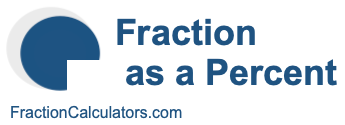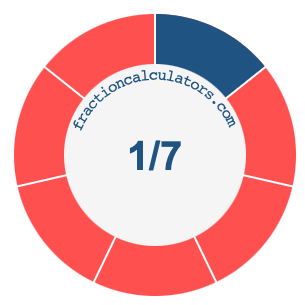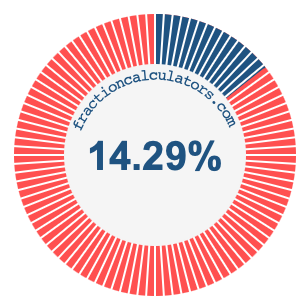1/7 as a percentWhat is 1/7 as a percent? Here we will explain and show you how to calculate and convert 1/7 to a percent.

In addition, we will also illustrate the fraction 1/7 on a pie chart and 1/7 as a percent on a pie chart.

1/7 is a fraction that means 1 out of 7, and percent means something out of 100. We can therefore make the following equation to get 1/7 as a percent:

 1 7
=
 percent 100

To find the percent in the equation above, we multiply 1 by 100 and then divide the product by 7. Here is the solution to the equation above and the answer to 1/7 as a percent:

 1 7
≈
 14.29 100

1/7 ≈ 14.29%

The illustrations below show you how 1/7 and 14.29% divide a pie chart differently, but cover the same area because they are the same.Fraction as a Percent Calculator
You can use this tool to convert another fraction to percent.

/

1/8 as a percent
Here is the next fraction on our list that we have converted to a percent for you.